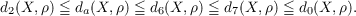#### Vol. 38, No. 1, 1971

 Download this articleFor screen For printingRecent Issues Vol. 325: 1  2 Vol. 324: 1  2 Vol. 323: 1  2 Vol. 322: 1  2 Vol. 321: 1  2 Vol. 320: 1  2 Vol. 319: 1  2 Vol. 318: 1  2Online Archive Volume: Issue:The Journal Subscriptions Editorial Board Officers Contacts Submission Guidelines Submission Form Policies for Authors ISSN: 1945-5844 (e-only) ISSN: 0030-8730 (print) Special Issues Author Index To Appear Other MSP Journals
Examples concerning sum properties for metric-dependent dimension functions

### John Charles Nichols and James C. Smith

Vol. 38 (1971), No. 1, 151–159
##### Abstract

Let d0 denote the metric dimension function defined by Katětov, and let dim be the covering dimension function. K. Nagami and J. H. Roberts introduced the metric-dependent dimension functions d2 and d3, and J. C. Smith defined the functions d6 and d7. The following relations hold for all metric spaces (X,ρ):Since all of the metric-dependent dimension functions above satisfy a “Weak Sum Theorem,” it is natural to ask if any of these functions satisfy the Finite Sum Theorem or the Countable Sum Theorem. In this paper the authors obtain new properties of these dimension functions, and using these results construct examples for which none of the metric dependent dimension functions satisfy either of the sum theorems in question.

Primary: 54F45
##### Milestones